Remainder Theoram

Chapter 2 Class 9 Polynomials
Concept wise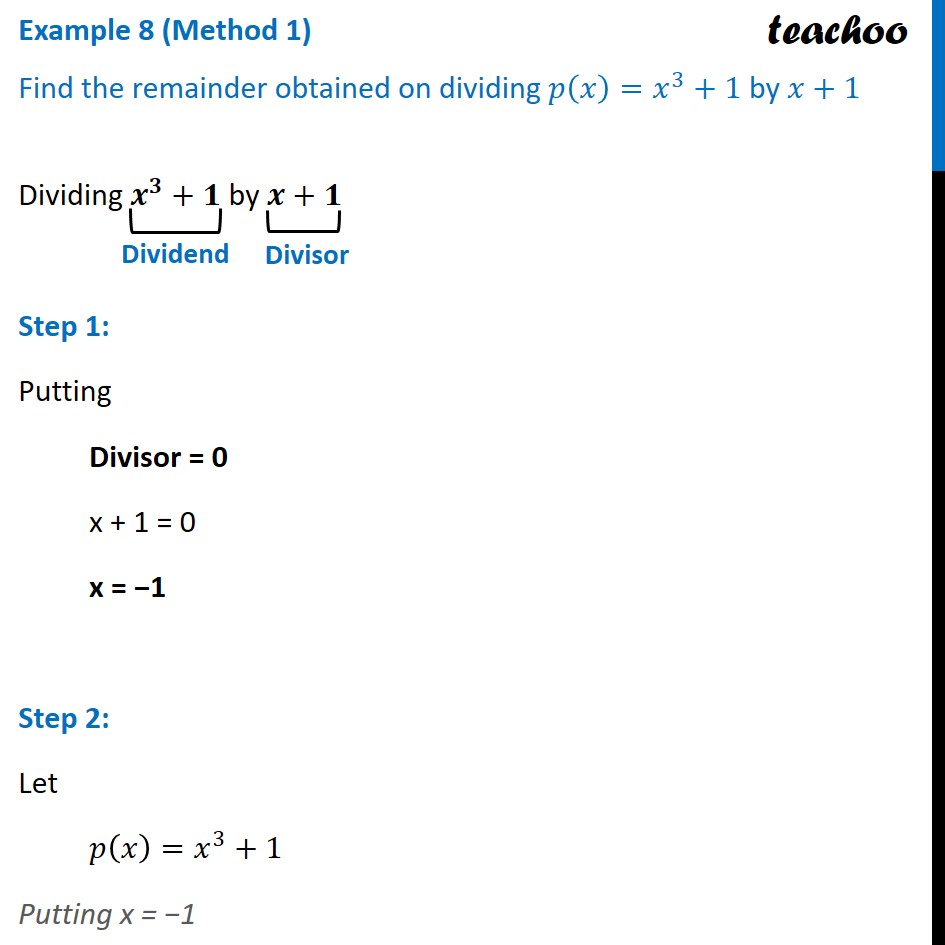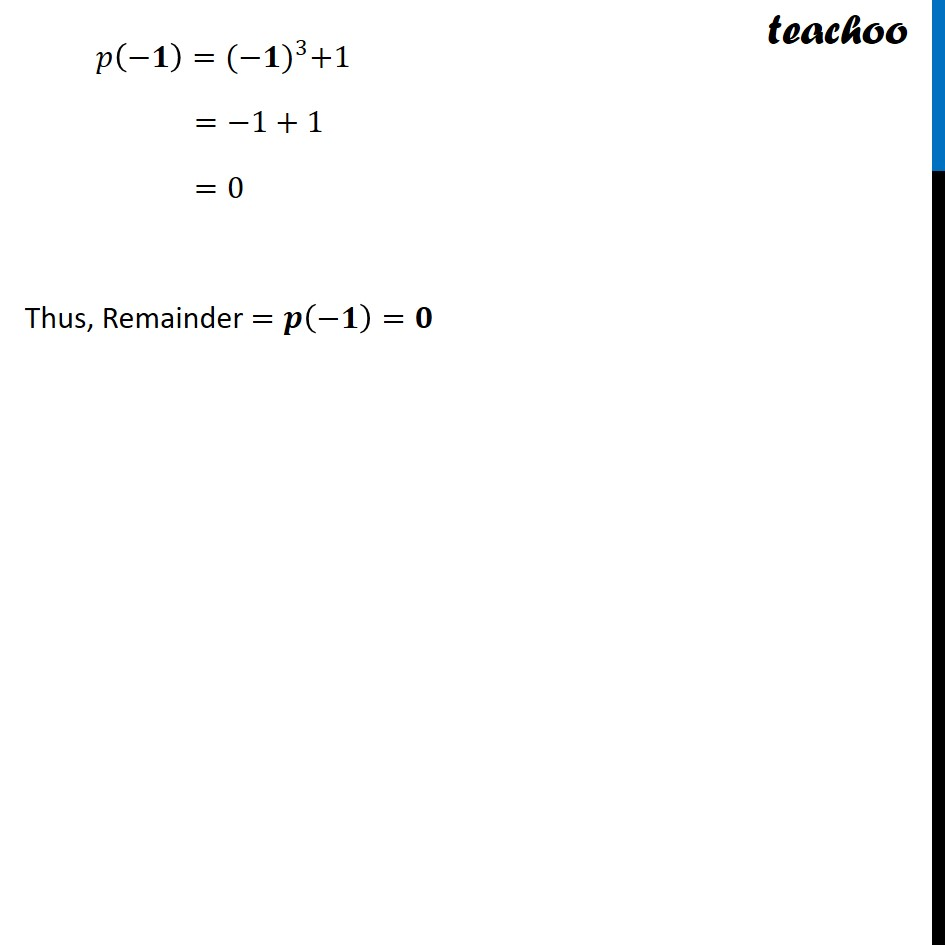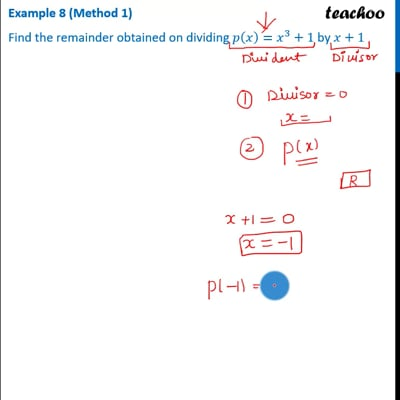This video is only available for Teachoo black users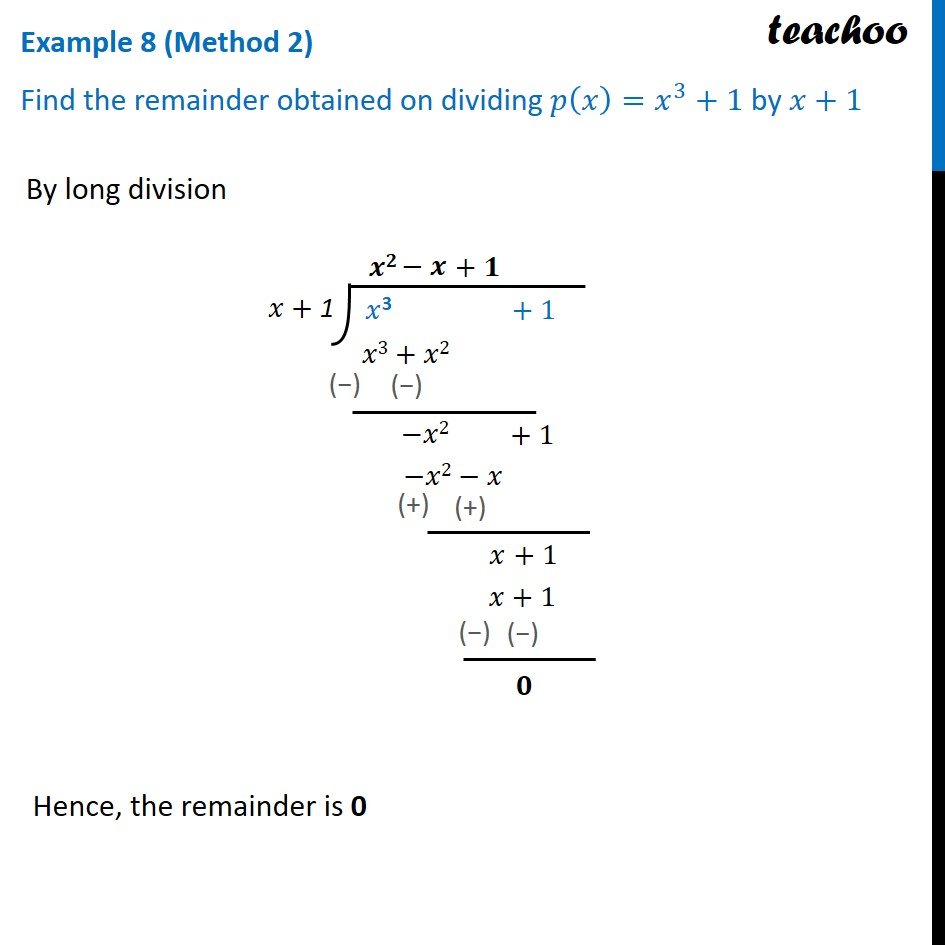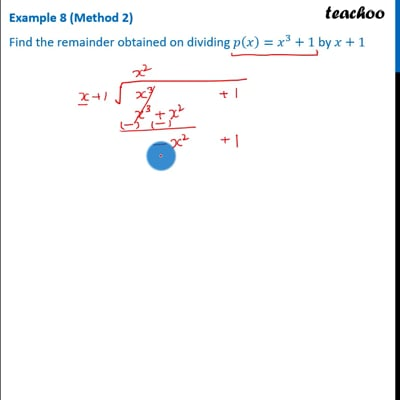This video is only available for Teachoo black users

Introducing your new favourite teacher - Teachoo Black, at only ₹83 per month

### Transcript

Example 8 (Method 1) Find the remainder obtained on dividing 𝑝(𝑥)=𝑥^3+1 by 𝑥+1 Dividing 𝒙^𝟑+𝟏 by 𝒙+𝟏 Step 1: Putting Divisor = 0 x + 1 = 0 x = −1 Step 2: Let 𝑝(𝑥)=𝑥^3+1 Putting x = −1 𝑝(−𝟏)=〖(−𝟏)〗^3+1 =−1+1 =0 Thus, Remainder =𝒑(−𝟏)=𝟎 Example 8 (Method 2) Find the remainder obtained on dividing 𝑝(𝑥)=𝑥^3+1 by 𝑥+1 By long division Hence, the remainder is 0